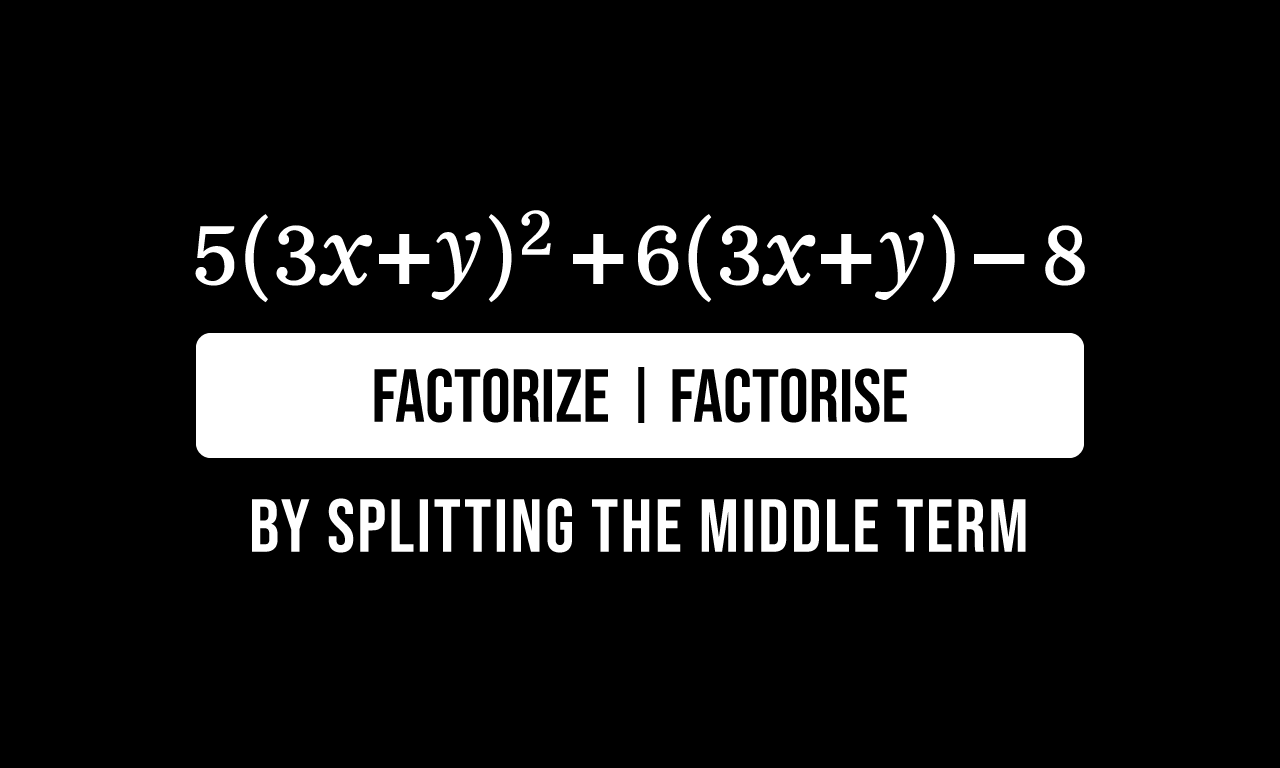# Factorize $5(3x+y)^2+6(3x+y)-8$ by splitting the middle termFive times the sum of three times $x$ and $y$ whole square plus six times sum of three times $x$ and $y$ minus eight is the given mathematical expression.

$5(3x+y)^2+6(3x+y)-8$

The given algebraic expression matches with the form of a quadratic expression but the factor $3x+4$ in first and second terms makes the algebraic expression complex.

### Simplify the Algebraic expression

It can be expressed to our known quadratic form for our convenience by representing the factor $3x+4$ by a variable. So, let’s denote the factor $3x+4$ by a variable $z$.

$=\,\,\,$ $5z^2+6z-8$

### Factorize the expression by splitting

Consider the first term $5z^2$ and the constant term $8$. Now, multiply both of them to find their product.

$5z^2 \times 8 \,=\, 40z^2$

The middle term is $6z$ in the given algebraic expression and let’s verify whether the product $40z^2$ can be split as either sum or difference of two terms, which should be equal to the middle term.

The product $40z^2$ can be split as a product of $10z$ and $4z$.

$\implies$ $40z^2 \,=\, 10z \times 4z$

The sum of the factors $10z$ and $4z$ is not equal to the middle term of the quadratic expression but their difference is equal to the middle term.

So, let’s split the middle term $6z$ as a difference of $10z$ and $4z$ in the algebraic expression.

$=\,\,\,$ $5z^2+10z-4z-8$

It is time to factorize the algebraic expression by grouping the terms.

$=\,\,\,$ $(5z^2+10z)-(4z+8)$

Now, let’s take the common factor out from the terms to factorise the algebraic expression.

$=\,\,\,$ $5z \times z$ $+$ $2 \times 5z$ $-$ $4z$ $-$ $8$

$=\,\,\,$ $5z \times (z+2)$ $-$ $4 \times z$ $-$ $4 \times 2$

$=\,\,\,$ $5z \times (z+2)$ $-$ $4 \times (z+2)$

$=\,\,\,$ $(z+2)(5z-4)$

### Bring back the Expression to actual form

The given algebraic expression is successfully factored but it is factored in terms of $z$. Actually, the algebraic expression is given in terms of a variable $x$. Hence, it should be converted in terms of $x$ from $z$.

$=\,\,\,$ $(3x+y+2)\big(5(3x+y)-4\big)$

$=\,\,\,$ $(3x+y+2)\big(5 \times (3x+y)-4\big)$

Now, distribute the factor $5$ over the addition of the terms $3x$ and $4$ as per the distributive property of multiplication over addition.

$=\,\,\,$ $(3x+y+2)(5 \times 3x+5 \times y-4)$

$=\,\,\,$ $(3x+y+2)(15x+5y-4)$

Latest Math Topics
Jun 26, 2023
Jun 23, 2023

###### Math Questions

The math problems with solutions to learn how to solve a problem.

Learn solutions

Practice now

###### Math Videos

The math videos tutorials with visual graphics to learn every concept.

Watch now

###### Subscribe us

Get the latest math updates from the Math Doubts by subscribing us.